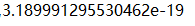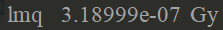# Doubts about units in Geant4

I recorded the dose deposited in the same volume using “UI-command base scoring” and the method in exampleB1, but the results confused me.This is the macro command file：

And the result_1 is(using “UI-command base scoring” ,I use “/score/quantity/get/unit” and it shows the unit is “Gy”):The result_2 is:The two results differ by exactly 10^12, why?

When you print out a quantity in Geant4, you need to (a) divide out the units, and (b) print the units you divded by. Geant4 internally uses a large collection of multipliers which together form a consistent set of unit. But all of the numerical quantities are simply doubles.

For a simple example, let’s store the radius of the Earth:

``````  G4double Rearth = 6380*km;
G4cout << Rearth << G4endl;
``````

The output will be

``````  6.38e+9
``````

What?!? That’s not the 6380 I put in! Internally Geant4 uses units where mm = 1. Consequently, km = 1e+6, with the result you see. The correct way to print out a variable with units is

``````  G4cout << "R(earth) = " << Rearth/km << " km" << G4endl;
``````

which reports

``````  R(earth) = 6380 km
``````

Just as we expect.

For your problem, we can ask why it’s off by 10^12. Well, let’s find out what the unit “gray” is, from `\$G4INCLUDE/CLHEP/Units/SystemOfUnits.h`:

``````  static constexpr double      gray = joule/kilogram ;
``````

and then we find (skipping “joule” for now),

``````  static constexpr double  kilogram = joule*second*second/(meter*meter);
``````

So we have gray = metermeter / (secondsecond). Geant4 uses units, as I noted above of mm=1 and ns=1. So gray = 1e31e3 / (1e91e9) = 1e6/1e18 = 1e-12. If you print out your result as

``````  G4cout << result_1/gray << " Gy" << G4endl;
``````

you’ll get 3.19e-19 / 1e-12 = `3.19e-7 Gy`, just as you expect.

I totally get it ！Thanks a lot for your serious and detailed answer. Best wishes！This topic was automatically closed 7 days after the last reply. New replies are no longer allowed.